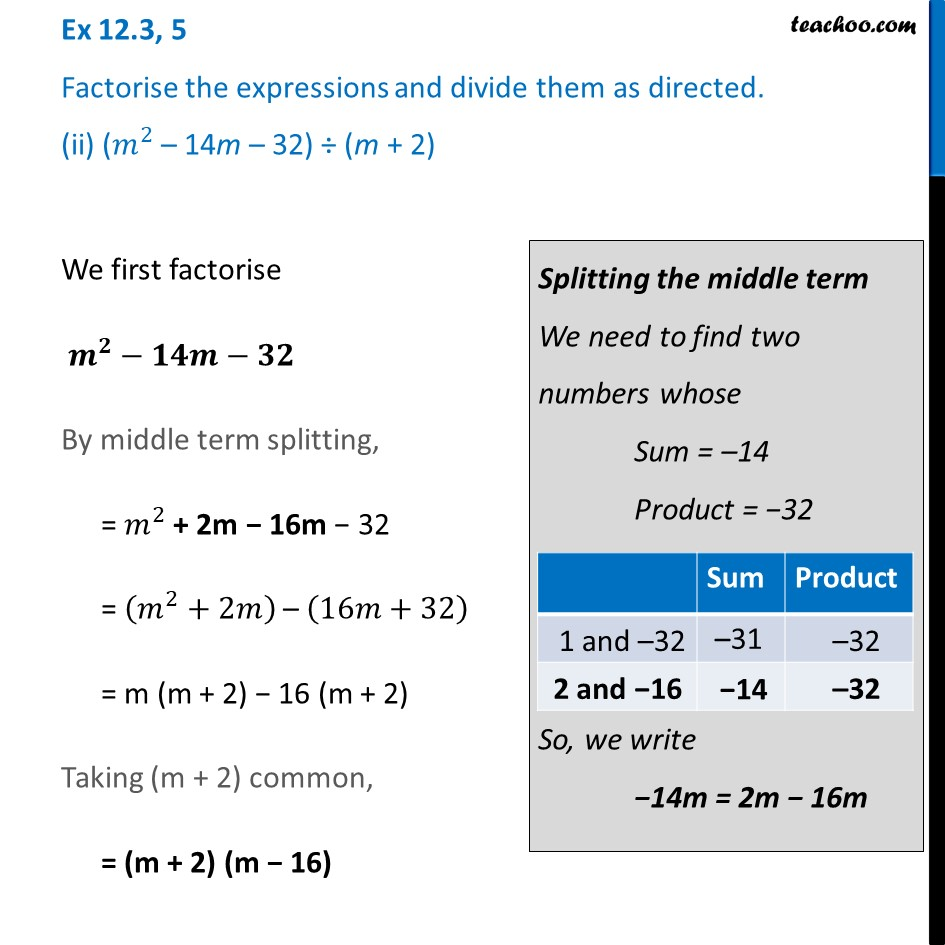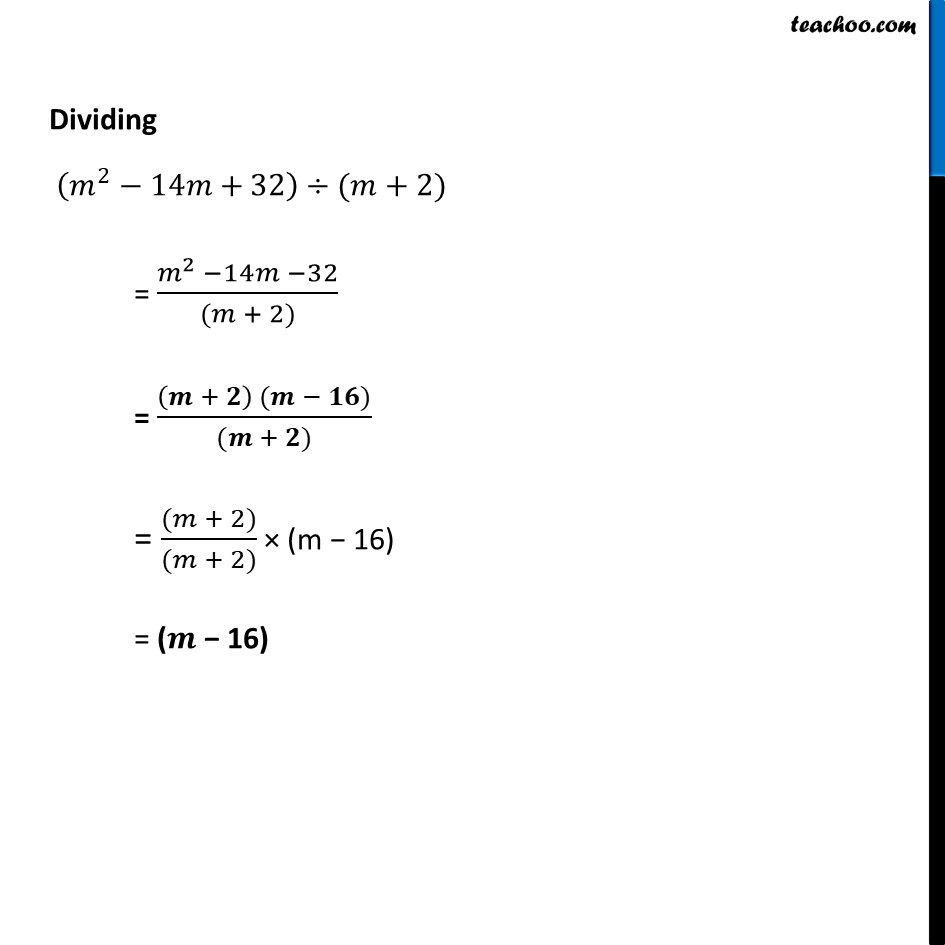Ex 12.3

Chapter 12 Class 8 Factorisation
Serial order wiseLearn in your speed, with individual attention - Teachoo Maths 1-on-1 Class

### Transcript

Ex 12.3, 5 Factorise the expressions and divide them as directed. (ii) (𝑚^2 – 14m – 32) ÷ (m + 2)We first factorise 𝒎^𝟐−𝟏𝟒𝒎−𝟑𝟐 By middle term splitting, = 𝑚^2 + 2m − 16m − 32 = (𝑚^2+2𝑚) – (16𝑚+32) = m (m + 2) − 16 (m + 2) Taking (m + 2) common, = (m + 2) (m − 16) Splitting the middle term We need to find two numbers whose Sum = –14 Product = −32 So, we write −14m = 2m − 16m Dividing (𝑚^2−14𝑚+32)÷(𝑚+2) = (𝑚^2 −14𝑚 −32)/((𝑚 + 2)) = ((𝒎 + 𝟐) (𝒎 − 𝟏𝟔))/((𝒎 + 𝟐)) = ((𝑚 + 2))/((𝑚 + 2)) × (m − 16) = (𝒎 − 16)